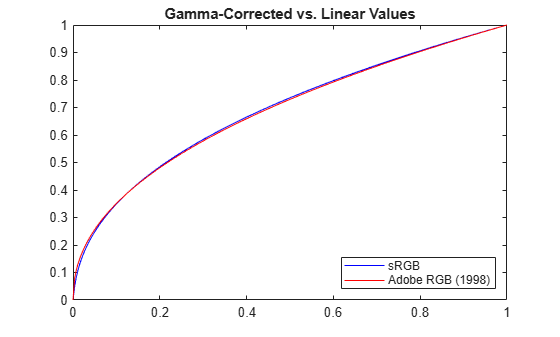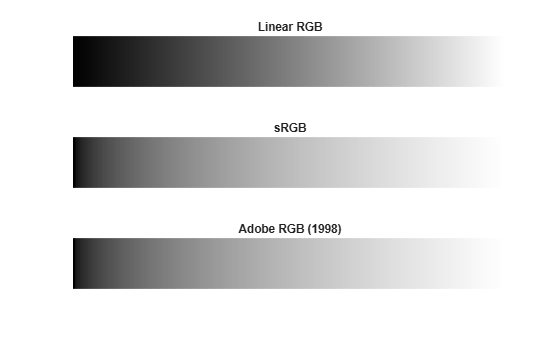# lin2rgb

Apply gamma correction to linear RGB values

## Syntax

``B = lin2rgb(A)``
``B = lin2rgb(A,Name,Value)``

## Description

example

````B = lin2rgb(A)` applies a gamma correction to the linear RGB values in image `A` so that `B` is in the sRGB color space, which is suitable for display.```

example

````B = lin2rgb(A,Name,Value)` applies gamma correction using name-value pairs to control additional options.```

## Examples

collapse all

Define a range of linear values. This vector defines 257 equally spaced points between 0 and 1.

`lin = linspace(0,1,257);`

Apply gamma correction to the linear values based on the sRGB standard. Then apply gamma correction to the linear values based on the Adobe RGB (1998) standard.

```sRGB = lin2rgb(lin); adobeRGB = lin2rgb(lin,'ColorSpace','adobe-rgb-1998');```

Plot the gamma-corrected curves.

```figure plot(lin,sRGB,'b',lin,adobeRGB,'r') title('Gamma-Corrected vs. Linear Values') legend('sRGB','Adobe RGB (1998)','Location','southeast')```For an alternative visualization, plot color bars representing each color space.

```cb_lin = ones(30,257) .* lin; cb_sRGB = ones(30,257) .* sRGB; cb_adobeRGB = ones(30,257) .* adobeRGB; figure subplot(3,1,1); imshow(cb_lin); title('Linear RGB') subplot(3,1,2); imshow(cb_sRGB); title('sRGB'); subplot(3,1,3); imshow(cb_adobeRGB); title('Adobe RGB (1998)');```The gamma-corrected color spaces get brighter more quickly than the linear color space, as expected.

Open an image file containing minimally processed linear RGB intensities.

`A = imread('foosballraw.tiff');`

The image data is the raw sensor data after correcting the black level and scaling to 16 bits per pixel. Interpolate the intensities to reconstruct color by using the `demosaic` function. The color filter array pattern is RGGB.

`A_demosaiced = demosaic(A,'rggb');`

Display the image. To shrink the image so that it appears fully on the screen, set the optional initial magnification to a value less than 100.

```figure imshow(A_demosaiced,'InitialMagnification',25) title('Sensor Data Without sRGB Gamma Correction')```The image appears dark because it is in the linear RGB color space. Apply gamma correction to the image according to the sRGB standard, storing the values in double precision.

`A_sRGB = lin2rgb(A_demosaiced,'OutputType','double');`

Display the gamma-corrected image, setting the optional magnification.

```figure imshow(A_sRGB,'InitialMagnification',25) title('Sensor Data With sRGB Gamma Correction');```The gamma-corrected image looks brighter than the linear image, as expected.

## Input Arguments

collapse all

Linear RGB color values, specified as a numeric array in one of the following formats.

• c-by-3 colormap. Each row specifies one RGB color value.

• m-by-n-by-3 image

• m-by-n-by-3-by-p stack of images

Data Types: `single` | `double` | `uint8` | `uint16`

### Name-Value Arguments

Specify optional pairs of arguments as `Name1=Value1,...,NameN=ValueN`, where `Name` is the argument name and `Value` is the corresponding value. Name-value arguments must appear after other arguments, but the order of the pairs does not matter.

Before R2021a, use commas to separate each name and value, and enclose `Name` in quotes.

Example: `B = lin2rgb(I,'ColorSpace','adobe-rgb-1998')` applies gamma correction to an image, `I`, according to the Adobe RGB (1998) standard.

Color space of the output image, specified as the comma-separated pair consisting of `'ColorSpace'` and `'srgb'` or `'adobe-rgb-1998'`.

Data Types: `char` | `string`

Data type of the output RGB values, specified as the comma-separated pair consisting of `'OutputType'` and `'double'`, `'single'`, `'uint8'`, or `'uint16'`. By default, the output data type is the same as the data type of `A`.

Data Types: `char` | `string`

## Output Arguments

collapse all

Gamma-corrected RGB image, returned as a numeric array of the same size as the input `A`.

## Algorithms

collapse all

### Gamma Correction Using the sRGB Standard

The gamma correction to transform linear RGB tristimulus values into sRGB tristimulus values is defined by the following parametric curve:

f(u) = -f(-u),             u < 0

f(u) = c ⋅ u,             0 ≤ u < d

f(u) = a ⋅ uɣ + b,      u ≥ d,

where u represents a color value with these parameters:

a = 1.055

b = –0.055

c = 12.92

d = 0.0031308

ɣ = 1/2.4

### Gamma Correction Using the Adobe RGB (1998) Standard

The gamma correction to transform linear RGB tristimulus values into Adobe RGB (1998) tristimulus values uses a simple power function:

v = uɣ,           u ≥ 0

v = -(-u)ɣ,      u < 0,

with

ɣ = 1/2.19921875

 Ebner, Marc. "Gamma Correction." Color Constancy. Chichester, West Sussex: John Wiley & Sons, 2007.

 Adobe Systems Incorporated. "Inverting the color component transfer function." Adobe RGB (1998) Color Image Encoding. Section 4.3.5.2, May 2005, p.12.